Published: 03 November 2014

# Bearing fault diagnosis based on TEO and SVM

Qingzhu Liu1
Yujie Cheng2
1, 2School of Reliability and Systems Engineering, Beihang University, Beijing 100191, China
1, 2Science and Technology on Reliability and Environmental Engineering Laboratory, Beijing 100191, China
Corresponding Author:
Qingzhu Liu
Views 23

#### Abstract

A fault method for bearing based on Teager energy operator (TEO) and support vector machine (SVM) is proposed in this paper. First, the total energy of the vibration signal of the bearing is estimated by the TEO technique, which has good time resolution for the instantaneous signal. Then, the Teager spectrums are obtained by applying fast Fourier transform (FFT) to the Teager energy signal. The feature frequencies of different fault modes, as well as the ratio of resonance frequency band energy to total energy in the Teager spectrum are extracted to form the feature vectors. Finally, these vectors are introduced into SVM to realize fault classification for the bearing. Experiments are conducted to verify the feasibility of the proposed method, the results show that the proposed method performs effectively to identify the failure mode of the bearing under variable conditions.

## 1. Introduction

Mechanics is becoming more and more important to the country with the development of the industry. There is no doubt that bearing, supporting shafts, is a critical component of rotating machinery and is easily damaged in the form of pitting corrosion, abrasion, erosion, rolling body injury, damage, and so on. If bearing is breakdown, it will cause enormous economic loss, influence industrial coherence and may even cause personal injury. So it is necessary to perform fault diagnosis for the bearing. Fortunately, many algorithms have been put forward to conduct analysis to the bearing fault.

Wavelet analysis and Empirical Mode Decomposition (EMD) have been used in signal processing and the feature extraction [1, 2]. The basic theory of wavelet analysis is to use a function to represent or approximate a signal. It has strong adaptive ability to the whole frequency domain. However, it is difficult to choose wavelet basis function. EMD can overcome this shortcoming and is adaptive to the change of signal, but it also has the disadvantage of end effect.

TEO can overcome all of the above shortcomings and be is available to the nonlinear signal. It has a high time resolution for the transient signal and output the total energy of the signal . Kaiser improved the TEO to process voice signals and the result is very good . Wang Tian-Jin used TEO to identify the characteristic frequency . Hou Guo-Ping proposed a new bearing fault detection method based on SVM . However, the training of neural networks needs large amount of data, which is hardly acquired in practice. Thus, SVM is proposed to realize fault classification for the bearing.

To verify the effectiveness of the proposed method, experiments have been conducted. The results show that the proposed method performs well in fault diagnosis for the bearing.

## 2. Methodology

### 2.1.1. TEO for continuous random signal

The TEO $\mathrm{\Psi }$ for continuous random signal is defined as :

1
$\mathrm{\Psi }\left(x\left(t\right)\right)=\left[\stackrel{˙}{x}\left(t\right){\right]}^{2}-x\left(t\right)\stackrel{¨}{x}\left(t\right),$

where $\stackrel{˙}{x}\left(t\right)$ and $\stackrel{¨}{x}\left(t\right)$ represent the first-order and the second-order derivative of $x\left(t\right)$ respectively.

Actually, the total energy of the vibration signal of the bearing is estimated by the TEO technique. Now the formula in Eq. (1) is proved with a realistic model. This model is an undamped system composed of a mass block whose quality is $m$ and a spring whose stiffness is $k$. According to Newton theorem, the motion equation of the mass is:

2
$m\stackrel{¨}{x}\left(t\right)+kx\left(t\right)=0,$

where $x\left(t\right)$ is displacement of the mass relative to the equilibrium position. The solution of the equation is:

3
$x\left(t\right)=A\mathrm{c}\mathrm{o}\mathrm{s}\left(\omega t+\phi \right),$
4
$\stackrel{˙}{x}\left(t\right)=-A\omega \mathrm{s}\mathrm{i}\mathrm{n}\left(\omega t+\phi \right),$
5
$\stackrel{¨}{x}\left(t\right)=-A{\omega }^{2}\mathrm{c}\mathrm{o}\mathrm{s}\left(\omega t+\phi \right),$

where $A$ is the vibration amplitude, $\omega$ is the inherent frequency, $\phi$ is the initial phase.

Then, mechanical energy is calculated, which is the sum of potential and kinetic:

6
$E=\frac{1}{2}k\left[x\left(t\right){\right]}^{2}+\frac{1}{2}m\left[\stackrel{˙}{x}\left(t\right){\right]}^{2}.$

Drive Eqs. (3), (4) into Eq. (6), get:

7
$E=\frac{1}{2}m{A}^{2}{\omega }^{2}.$

Now, use this model to solve the instantaneous energy output of the TEO. Substitute Eqs. (3), (4), (5) into Eq. (1), get:

8
$\mathrm{\Psi }\left(x\left(t\right)\right)=\mathrm{\Psi }\left(A\mathrm{c}\mathrm{o}\mathrm{s}\left(\omega t+\phi \right)\right)={A}^{2}{\omega }^{2}.$

In contrast to Eq. (7), (8) missed $m/\text{2}$. So we can conclude that TEO track the total energy of simple harmonic motion signal ($m/$2 is a constant).

### 2.1.2. TEO for discrete signal

TEO $\mathrm{\Psi }$ for discrete signal is defined as:

9
$\mathrm{\Psi }\left(x\left(n\right)\right)=\left[x\left(n\right){\right]}^{2}-x\left(n-1\right)x\left(n+1\right).$

The energy of the signal only needs three data which can be calculated at any moment by TEO. So TEO have good time resolution for the instantaneous signal, and can detect transient component of signal.

### 2.2. SVM

The main idea of SVM is as shown in Fig. 1 . The triangles represent one kind of samples, while circles represent another. H represents the classifying line. H1, H2 are lines parallel to H and they are through the samples which are the closest to H at the same time. The distance between H1 and H2 is defined as class interval. The role of H is to correctly separate two kinds of samples and reaches zero of training error rate. The optimal classifying line is reaching classification margin maximization in Fig. 1.

The equation for classification line is:

10
$\omega \cdot x+b=0.$

Eq. (10) uses normalized treatment and gets linearly separable data sets$\left({x}_{i},{y}_{i}\right)\text{,}$$\left(i=\text{1,}\text{}\text{2,...}\text{,}\text{}n\right)$, $x\in {R}^{d}$, $y\in \left\{$1, –1$\right\}$ meet the following definitions

11
${y}_{i}\left({\omega }_{i}\cdot x+b\right)\ge 1,i=\text{1,}\text{}\text{2,...,}\text{}n.$

2$/‖\omega ‖$ is class interval. The classification margin maximization is the minimum of ${‖\omega ‖}^{2}$. The optimal classification line is to minimize the ${‖\omega ‖}^{2}/$2 and meats the type Eq. (11). Support vector is the training samples on H1 and H2.

SVM is developed for a value of two proposed classification problems. However, the bearing fault classification is a multi-class classification problem. So the multi-class classification problem should be converted to two classification problems . This experiment adopts a multi-classifier made of three SVMs.

Fig. 1Optimal classifying line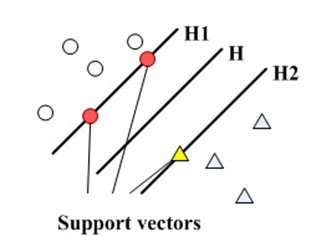Fig. 2The flow chart of the proposed method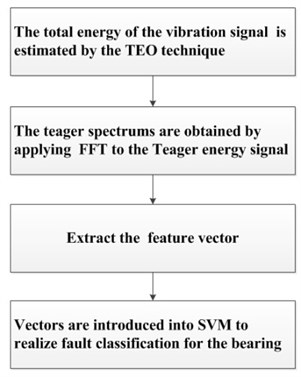### 2.3. The procedure of the proposed method

This paper proposes a fault diagnosis method for bearing based on TEO and SVM. First, the total energy of the vibration signal of the bearing is estimated by the TEO technique. Second, the teager spectrums are obtained by applying fast Fourier transform (FFT) to the Teager energy signal [10, 11]. Third, feature vectors are extracted. Finally, these vectors are introduced into SVM to realize fault classification for the bearing.

## 3. Case study

### 3.1. Feature extraction

All the rolling bearing data used in this study are acquired by the Bearing Data Center of Case Western Reserve University. In the experiment, natural frequency of bearing used in this paper is ${F}_{In}=$157.94 Hz, ${F}_{Out}=$104.56 Hz.

The total energy of the vibration signal of the bearing is estimated by the TEO technique. The time domain – amplitude waveforms are obtained as shown in Fig. 3 by inputting normal data, inner fault data and outer fault data to Teager program, respectively.

In order to obtain Teager spectrum, this paper uses FFT to transform TEO, the results are shown in Fig. 4. Resonance frequency band energy ${E}_{1}$ is the energy from 2000 to 4000 Hz. Because energy leakage is inevitable. According to the practice that $\mathrm{\Delta }f=$2 Hz, this experiment chooses $E\left({F}_{IN}±2\right)$, $E\left({F}_{OUT}±2\right)$, and ${E}_{1}/{E}_{TOTAL}$ as the feature vectors.

Fig. 3Time domain waveform at outer ring fault condition, inner fault condition, normal condition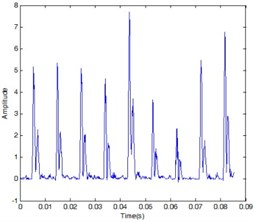a)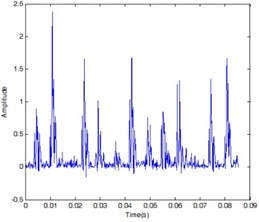b)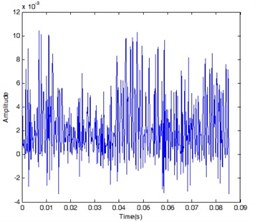c)

Fig. 4Frequency spectrum at outer ring fault condition, inner fault condition, normal condition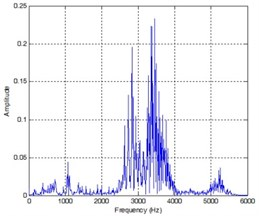a)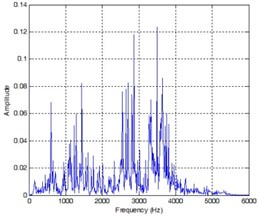b)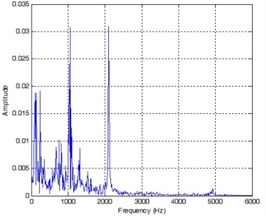c)

Table 1Feature vectors

 Inner ring (Hz) Outer ring (Hz) Ratio of energy (Hz) Feature vectors $E\left({F}_{IN}±2\right)$ $E\left({F}_{OUT}±2\right)$ ${E}_{1}/{E}_{TOTAL}$

Table 2Bearing fault identification

 $E\left({F}_{IN}±2\right)$ $E\left({F}_{OUT}±2\right)$ ${E}_{1}/{E}_{TOTAL}$ $SVM1$ $SVM2$ $SVM3$ Outer 4.6848 0.8221 0.0567 1 Correct Ring 4.6823 0.8210 0.0564 1 Correct Fault 4.6817 0.8201 0.0565 1 Correct Inner 0.3234 0.8723 0.1538 0 1 Correct Ring 0.3239 0.8721 0.1538 0 1 Correct Fault 0.3236 0.8724 0.1538 0 1 Correct Normal 0.0005 0.0005 0.1759 0 0 1 Correct 0.0006 0.0005 01756 0 0 1 Correct 0.0007 0.0005 0.1758 0 0 1 Correct

### 3.2. Fault diagnosis based on SVM

This experiment adopts a multi-classifier composed of three SVMs. The experiment uses a total of 20×3 groups data (each mode is 20 groups) to classify the faults. Set the targets output as Eq. (1), (0, 1) and (0, 0, 1), corresponding to the outer ring fault, the inner ring fault, and the normal condition, respectively. Because the place is limited, only parts of data (3×3 groups) is showed in Table 2.

## 4. Conclusions

This paper proposes a fault diagnosis method for bearing based on TEO and SVM. Experiments have been conducted to verify the effectiveness of the proposed method. From the results it can be seen that: 1) TEO can be used to calculate the total energy of signal, and have a good time resolution. 2) SVM is suitable to diagnose and is extremely accurate as well. 3) The proposed method has a good adaptability, and high accuracy.